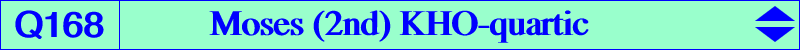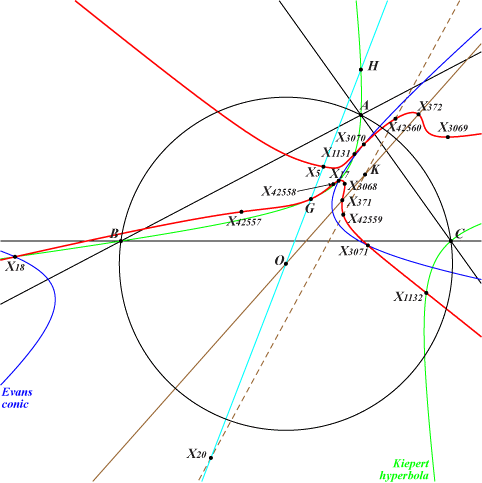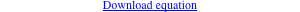too complicated to be written here. Click on the link to download a text file.X(2), X(5), X(17), X(18), X(371), X(372), X(1131), X(1132), X(3068), X(3069), X(3070), X(3071), X(8976), X(13951), X(31412), X(39641), X(39642), X(42557), X(42558), X(42559), X(42560), X(42561) X(39641), X(39642) are the imaginary foci of the Brocard inellipse, on the Brocard axis and the Kiepert hyperbolaQ168 is a KHO-curve, see K1191 and CL075 for information. See also Q073 and Q167, two other KHO-quartics. The KHO-equation is : 2x^4 + 3x^2 y (10y - 9z) - 9 (y -z) (2y - z) (6y^2 - 3yz + 2z^2) = 0.